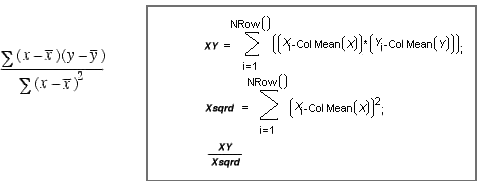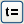Publication date: 05/24/2021

## Use Local Variables Formulas

Suppose you want to compute the slope in a simple linear regression of y on x using the standard formula shown in Figure 7.21. One way to do this is to create two local variables, and name them XY and Xsqrd. (See Use Local Variables in Formulas.) Then assign them to the numerator and the denominator calculations of the slope formula. Delimit each assignment with a semicolon, as shown in Figure 7.21. (Statements in the Formula Editor are actually JSL programming statements. Multiple statements in a formula must be separated by a semicolons.) The slope computation is simplified to XY divided by Xsqrd.

Figure 7.21 Local Variables in a Standard Slope FormulaNote: You can also create local variables using thebutton on the on-screen keypad. Clicking this button creates a local variable with a default name in an expression and places a semicolon after it. The default name is t1, and additional local variables are named t2, t3, and so on. You can change these default names by double-clicking and editing the name. However, you must be careful to rename each instance of the variable to avoid errors.